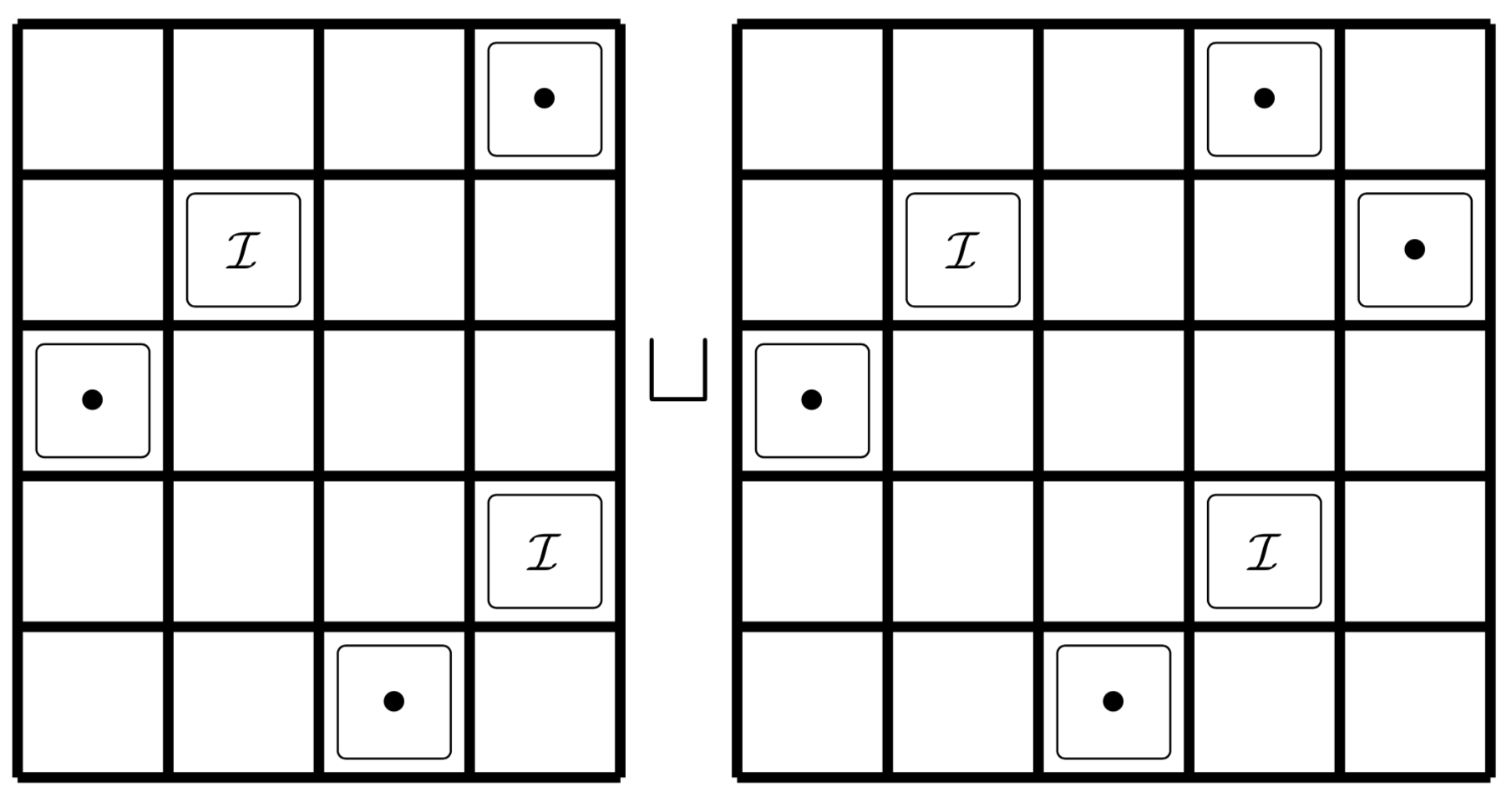# Automatic discovery of structural rules of permutation classes

### Mathematics of Computation, Volume 88, Number 318, July 2019, Pages 1967–1990We introduce an algorithm that produces conjectures regarding the structure of permutation classes. These conjectures take the form of a disjoint cover of “rules”, each of which is similar to a generalized grid class. Such a cover is usually easily verified by a human and can often be translated directly into an enumeration. The algorithm is successful in many cases where other algorithms fail and is theoretically guaranteed to succeed on any polynomial permutation class. We apply it to every non-polynomial permutation class avoiding a set of length four patterns. The structures found by the algorithm sometimes allow one to enumerate a permutation class with respect to permutation statistics, and often yield a method for sampling uniformly at random from the class. We additionally sketch a second algorithm formalizing the human verification of the conjectured covers.

Some of this work can be found in Christian’s thesis which can be found below.